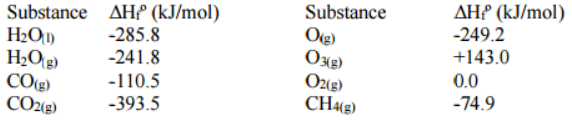# Problem: Given the standard enthalpies of formation in the table below, calculate the standard enthalpy change (ΔH°) in KJ for the following reaction.CH4(g) + O3(g) → CO2(g) + 2H2O(g)a. -2979KJb. 2979KJc. -703KJd. 703KJ e. -853KJ

###### FREE Expert Solution
89% (350 ratings)
###### Problem Details

Given the standard enthalpies of formation in the table below, calculate the standard enthalpy change (ΔH°) in KJ for the following reaction.

CH4(g) + O3(g) → CO2(g) + 2H2O(g)

a. -2979KJ

b. 2979KJ

c. -703KJ

d. 703KJ

e. -853KJWhat scientific concept do you need to know in order to solve this problem?

Our tutors have indicated that to solve this problem you will need to apply the Enthalpy of Formation concept. You can view video lessons to learn Enthalpy of Formation. Or if you need more Enthalpy of Formation practice, you can also practice Enthalpy of Formation practice problems.

What is the difficulty of this problem?

Our tutors rated the difficulty ofGiven the standard enthalpies of formation in the table belo...as medium difficulty.

How long does this problem take to solve?

Our expert Chemistry tutor, Dasha took 4 minutes and 50 seconds to solve this problem. You can follow their steps in the video explanation above.

What professor is this problem relevant for?

Based on our data, we think this problem is relevant for Professor Dunning's class at TEXAS.